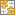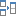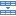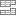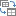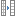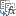# Excel IF wildcard statement for partial text match

Trying to build an IF statement with wildcard text, but it fails every time? The problem is not in your formula but in the function itself - Excel IF does not support wildcard characters. However, there is a way to get it to work for partial text match, and this tutorial will teach you how.

Whenever you want to perform partial or fuzzy matching in Excel, the most obvious solution is to use wildcards. But what if a specific function that you need to use does not support wildcards characters? Sadly, Excel IF is one of such functions. This is especially disappointing considering that other "conditional" functions such as COUNTIF, SUMIF, and AVERAGEIFS work with wildcards perfectly well.

Luckily, it is not the obstacle that can stop a creative Excel user :) By combining IF with other functions, you can force it to evaluate a partial match and get a nice alternative to an Excel IF wildcard formula.

## Why Excel IF function with wildcard not working

In the sample table below, supposing you want to check whether the IDs in the first column contain the letter "A". If found - display "Yes" in column B, if not - display "No".

It seems like including wildcard text in the logical test would be an easy solution:

`=IF(A2="*a*","Yes", "No")`

But regrettably it does not work. The formula returns "No" for all the cells, even those that contain "A":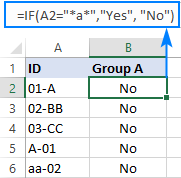Why does a wildcard IF statement fail? From all appearances, Excel doesn't recognize wildcards used with an equal sign or other logical operators. If you take a close look at the list of functions supporting wildcards, you will notice that their syntax assumes a wildcard text to appear directly in an argument like:

`=COUNTIF(A2:A10, "*a*")`

## Excel IF statement with wildcard text

Now that you know the reason why a wildcard IF formula fails, let's try to figure out how to get it to work. For this, we'll simply embed a function that accepts wildcards in the logical test of IF, namely the COUNTIF function:

IF(COUNTIF(cell, "*text*"), value_if_true, value_if_false)

With this approach, IF has no problem with understanding wildcards and flawlessly identifies the cells that contain either "A" or "a" (since COUNTIF is not case-sensitive):

`=IF(COUNTIF(A2, "*a*"),"Yes", "No")`

This formula goes to B2, or any other cell in row 2, and then you can drag it down to as many cells as needed: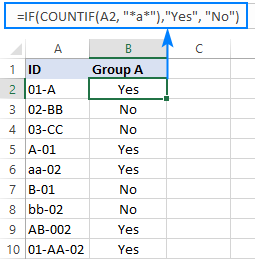This solution can also be used to locate strings of a specific pattern. Assuming only the IDs consisting of 2 groups of 2 characters separated with a hyphen are valid, you can use the "??-??" wildcard string to identify them:

`=IF(COUNTIF(A2, "??-??"), "Valid", "")`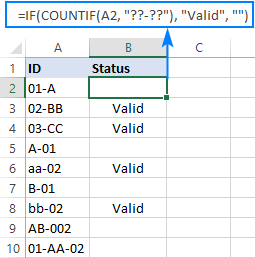How this formula works:

For the logical test of IF, we use the COUNTIF function that counts the number of cells matching the specified wildcard string. Since the criteria range is a single cell (A2), the result is always 1 (match is found) or 0 (match is not found). Given that 1 equates to TRUE and 0 to FALSE, the formula returns "Valid" (value_if_true) when the count is 1 and an empty string (value_if_false) when the count is 0.

## IF ISNUMBER SEARCH formula for partial matches

Another way to force Excel IF to work for partial text match is to include either the FIND or SEARCH function in the logical test. The difference is that FIND is case-sensitive while SEARCH is not.

So, depending on whether you want to treat lowercase and uppercase as the same or different characters, one of these formulas will work a treat:

Case-insensitive formula for partial match:

IF(ISNUMBER(SEARCH("text", cell)), value_if_true, value_if_false)

Case-sensitive formula for partial match:

IF(ISNUMBER(FIND("text", cell)), value_if_true, value_if_false)

As both functions are designed to perform a "cell contains" type of match, wildcards aren't really needed in this case.

For example, to detect IDs containing "A" or "a", the formula is:

`=IF(ISNUMBER(SEARCH("A", A2)), "Yes", "No")`

To only search for a capital "A" and ignore "a", the formula is:

`=IF(ISNUMBER(FIND("A", A2)), "Yes", "No")`

In B6 in the screenshot below, you can observe the difference in the result: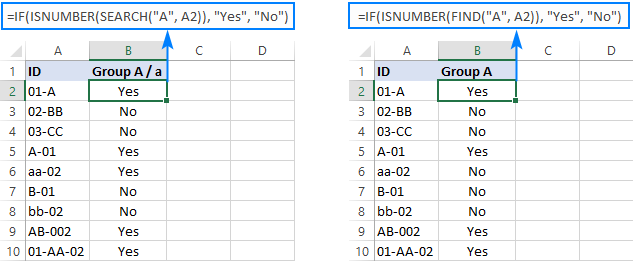How this formula works:

At the heart of the formula, there is a combination of ISNUMBER and SEARCH (or FIND):

`ISNUMBER(SEARCH("A", A2))`

The SEARCH function looks for the specified text ("A" in this example) and returns its position within a string in A2. If the text is not found, a #VALUE error is returned. As both SEARCH and FIND are designed to perform a "cell contains" type of match, wildcards aren't really needed in this case.

The ISNUMBER function converts a number to TRUE and any other value including error to FALSE. The logical value goes directly to the logical test of IF. In our case, A2 contains "A", so ISNUMBER returns TRUE:

`IF(TRUE, "Yes", "No")`

As the result, IF returns the value set for the value_if_true argument, which is "Yes".

## Excel IF OR statement with wildcards

Need to identify cells that contain one of wildcard text strings? In this case, you can combine the classic IF OR statement with the COUNTIF or ISNUMBER SEARCH formula discussed above.

For example, to search for "aa" OR "bb" in A2 ignoring the letter case and return "Yes" if either is found, use one of these formulas:

`=IF(OR(ISNUMBER(SEARCH("aa", A2)), ISNUMBER(SEARCH("bb", A2))), "Yes", "")`

or

`=IF(OR(COUNTIF(A2, "*aa*"), COUNTIF(A2, "*bb*")), "Yes", "")`

Adding up two COUNTIF functions will also work. In this case, the plus sign works like the OR operator:

`=IF(COUNTIF(A3, "*aa*") + COUNTIF(A3, "*bb*"), "Yes", "")`

Instead of hardcoding wildcard strings in the formula, you can input them in separate cells, say D2 and F2, as shown in the screenshot below. Please notice that these cell references are locked with the \$ sign so that the formula copies correctly to the below cells:

`=IF(OR(COUNTIF(A2, "*"&\$D\$2&"*"), COUNTIF(A2, "*"&\$F\$2&"*")), "Yes", "")`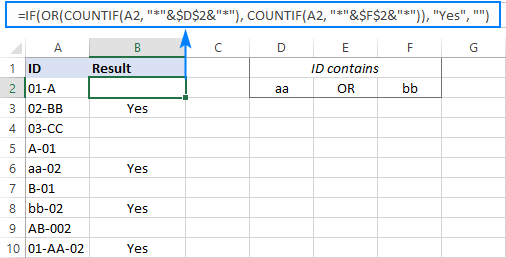The above formulas work well for 2 partial matches, but if you are searching for 3 or more, they would become too lengthy. In this case, it stands to reason to approach the task differently:

Supply multiple substrings to the SEARCH function in an array constant, count the returned numbers, and check if the result is greater than zero (which would mean that at least one of the substrings if found):

`=IF(COUNT(SEARCH({"aa","bb"}, A2))>0, "Yes", "")`

This way, you will get exactly the same result with a more compact formula: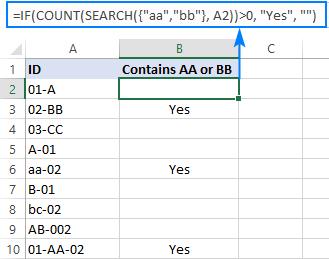## Excel IF AND formula with wildcards

When you want to check if a cell contains two or more different substrings, the easiest way is to use the COUNTIFS function with wildcards for the logical test.

Supposing you want to locate cells in column A that contain both "b" AND "2". To have it done, use "*b*" and "*2*" for COUNTIFS's criteria and A2 for the criteria range:

`=IF(COUNTIFS(A2, "*b*", A2, "*2*"), "Yes", "")`

Another way is to use the IF AND formula together with ISNUMBER SEARCH:

`=IF(AND(ISNUMBER(SEARCH("b", A2)), ISNUMBER(SEARCH("2", A2))), "Yes", "")`

Though we do not include any wildcard characters in this formula, it does work like searching for two wildcard strings ("*b*" and "*2*") in the same cell.

Of course, nothing prevents you from entering the search values in predefined cells, D2 and F2 in our case, and supplying the cell references to the formula:

`=IF(AND(ISNUMBER(SEARCH(\$D\$2, A2)), ISNUMBER(SEARCH(\$F\$2, A2))), "Yes", "")`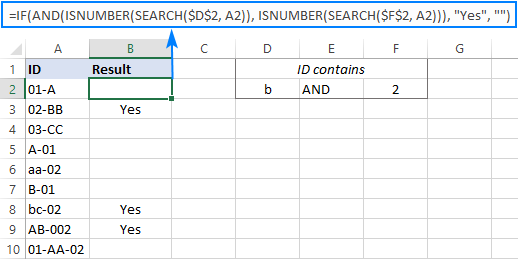If you prefer using more compact formulas wherever possible, then you may better like the array constant approach. The IF COUNT SEARCH formula is very much like in the previous example, but because this time both substrings must appear in A2, we check if the count is equal to 2:

`=IF(COUNT(SEARCH({"b","2"}, A2))=2, "Yes", "")`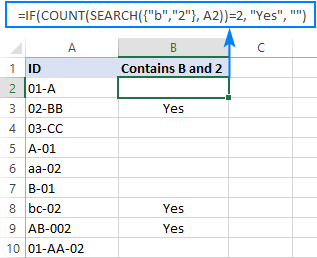These are the main methods of using wildcard in IF statement in Excel. If you know any other solutions, other users will certainly appreciate if you share your experience in comments. I thank you for reading and hope to see you on our blog next week!